Courses

# JEE Notes | EduRev

## JEE: JEE Notes | EduRev

The document JEE Notes | EduRev is a part of the JEE Course Chemistry for JEE.
All you need of JEE at this link: JEE

Early Models of Periodic Table

Classification of Elements was necessary since many elements were being discovered in the 19th century and the study of these elements individually was proving difficult.

There were many attempts at classifying elements including ‘Dobereiner’s Triads’ and ‘Newland’s Octaves’.

• German chemist Johann Wolfgang Dobereiner attempted to classify elements with similar properties into groups of three elements each. These groups were called ‘triads’.
• Dobereiner suggested that in these triads, the atomic mass of the element in the middle would be more or less equal to the mean of the atomic masses of the other two elements in the triad.
• An example of such a triad would be one containing lithium, sodium, and potassium. The atomic mass of lithium 6.94 and that of potassium is 39.10. The element in the middle of this triad, sodium, has an atomic mass of 22.99 which is more or less equal to the mean of the atomic masses of lithium and potassium (which is 23.02).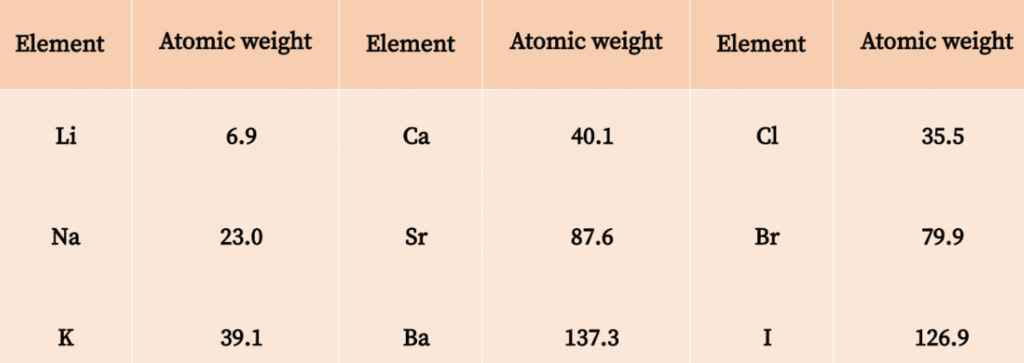The Limitations of Dobereiner’s Triads are:

• All the elements known at that time couldn’t be classified into triads.
• Only four triads were mentioned – (Li, Na, K+Ca, Sr, Ba+, Cl, Br, I+, S, Se, Te).

Newland’s Octaves

• English scientist John Newlands arranged the 56 known elements in increasing order of atomic mass in the year 1866.
• He observed a trend wherein every eighth element exhibited properties similar to the first. This similarity in the properties of every eighth element can be illustrated as follows.
• Newland’s Law of Octaves states that when the elements are arranged in increasing order of atomic mass, the periodicity in properties of two elements which have an interval of seven elements in between them would be similar.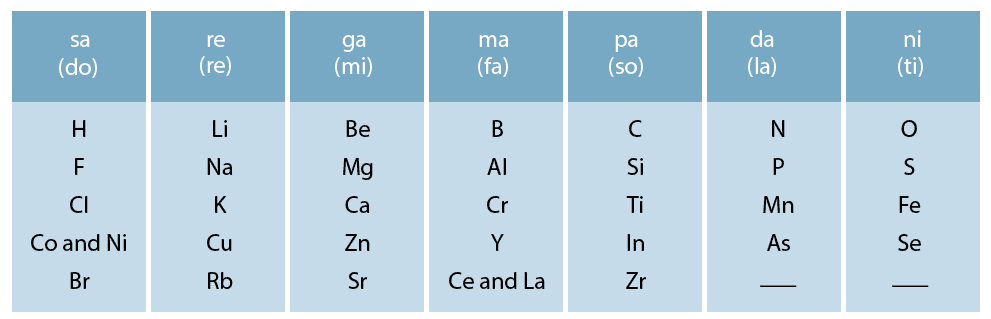Limitations of Newland’s octaves are:

1. It was only up till calcium that the classification of elements is done via Newland’s Octaves.
2. The discovery of noble gases added to the limitations of this method since they couldn’t be included in this arrangement without disturbing it completely.

Mendeleev’s Periodic Table and Law

• Russian chemist Dmitri Ivanovich Mendeleev put forth his periodic table in 1869. He observed that the properties of elements, both physical and chemical, were periodically related to the atomic mass of the elements.
• The Periodic Law (also referred to as Mendeleev’s Law), states that the chemical properties of elements are a periodic function of their atomic weights.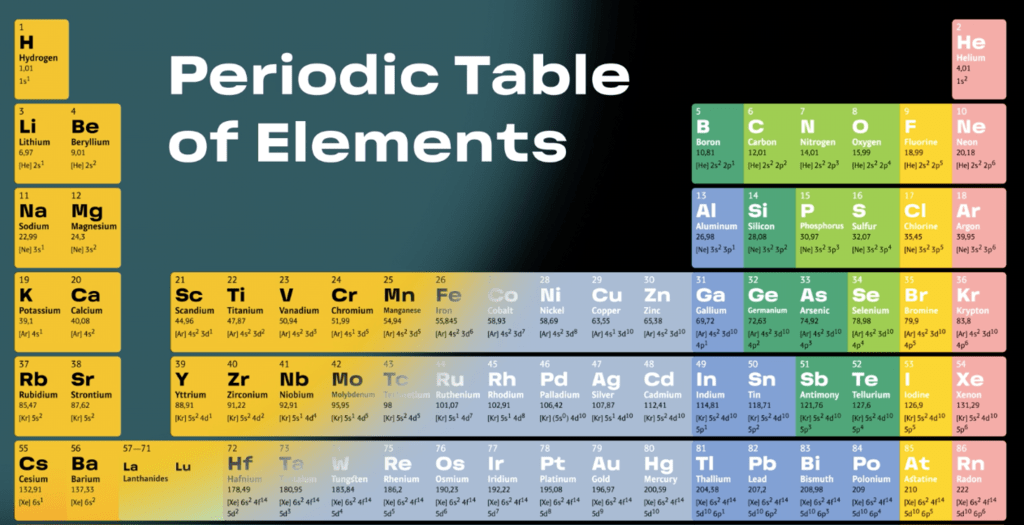Advantages of Mendeleev’s Periodic table are:

1. The inclusion of these newly discovered elements did not disturb the periodic table. Examples include germanium, gallium, and scandium.
2. It was used to correct the wrong atomic weights in use at that time.
3. A variance from the atomic weight order was provided by Mendeleev’s table.

Limitations of Mendeleev’s Periodic table are:

1. Hydrogen’s position was in the group of alkali metals but hydrogen also exhibited halogen like qualities.
2. Isotopes were positioned differently since this type of classification of elements was done by considering the atomic weight of the element. Therefore – protium, deuterium, and tritium would occupy varying positions in Mendeleev’s table.
3. An anomalous positioning of a few elements showed that the atomic masses did not increase regularly from one element to the next. An example of this would be the placement of cobalt (atomic mass of 58.9) before nickel (atomic mass of 58.7).

Modern Periodic Law

“The physical and chemical properties of the elements are periodic functions of their atomic numbers”.

• Properties of elements are the periodic function to their atomic numbers.
• The periodicity in properties is due to the repetition of similar outer shell electronic configuration at certain regular intervals.
• In the modern periodic table is based on modern periodic law in which elements are arranged in increasing order of their atomic numbers.
• In the modern periodic table, the elements are arranged in rows and columns. These rows and columns are known as periods and groups respectively.
• The table consists of 7 periods and 18 groups
• The period indicates the value of ‘n’ (principal quantum number) for the outermost or valence shell.
• The same number of electrons is present in the outer orbitals (that is, similar valence shell electronic configuration

Classification of Elements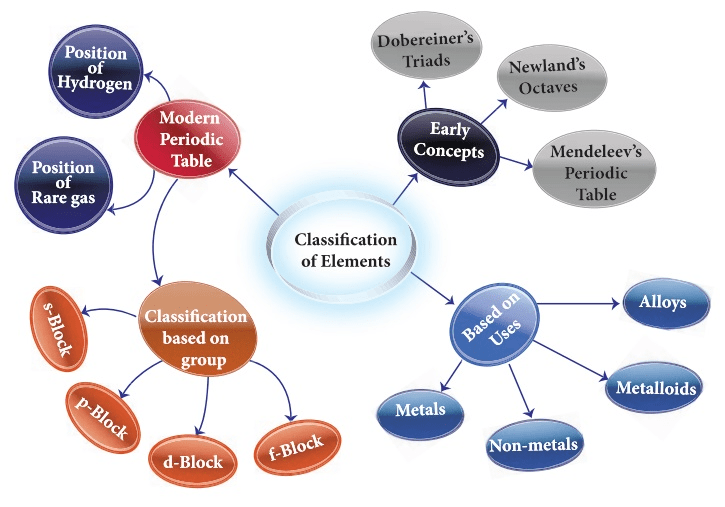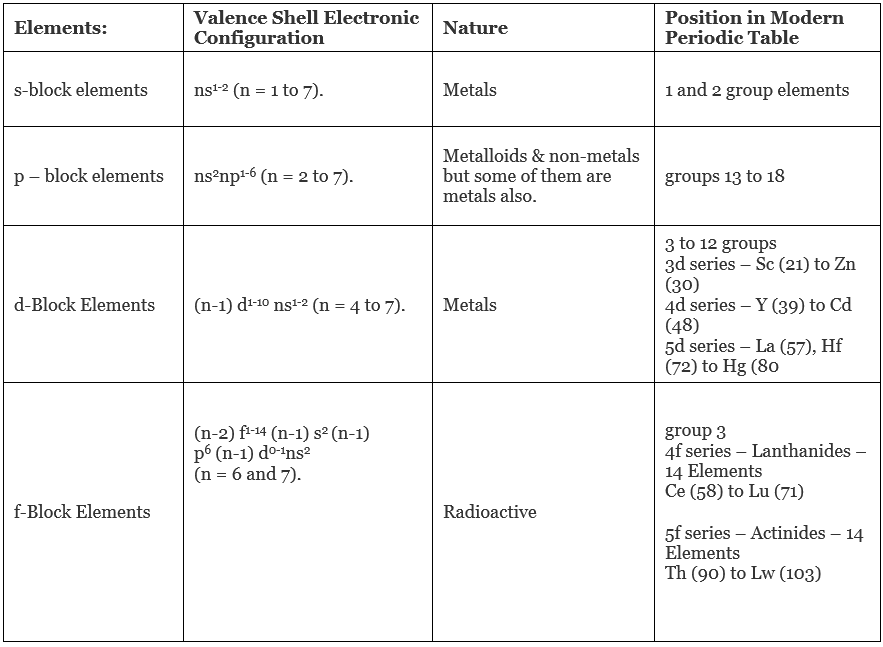Periodicity in Atomic Properties

• Within a given period atomic radius decreases from left to right. This is due to the effect of increase in nuclear charge while the electrons are being added to the same shell.
• Within a given group atomic radius increases down the group. This is due to the increase in number of shells.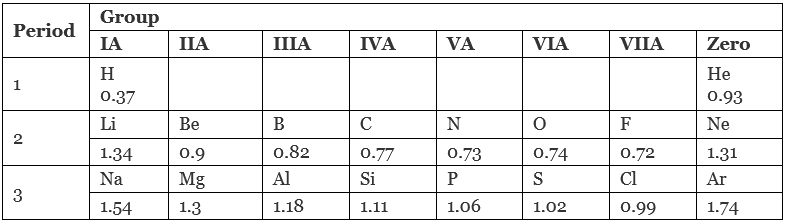• In the first transition series the atomic size slightly decreases from Sc to Mn because effect of effective nuclear charge is stronger than the shielding effect. The atomic size from Fe to Ni remains almost the same because both the effects balance each other.
• The atomic size from Cu to Zn slightly increases because shielding effect is more than effective nuclear charge due to d10 structure of Cu and Zn.
• Inner transition elements – As we move along the lanthanide series, there is a decrease in atomic as well as ionic radius. The decrease in size is regular in ions but not so regular in atoms. This is called lanthanide contraction.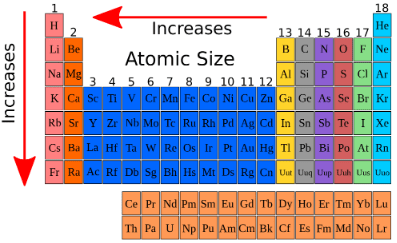2. Ionisation potential or Ionisation Energy

Ionization energy increases along the period while decreases down the group.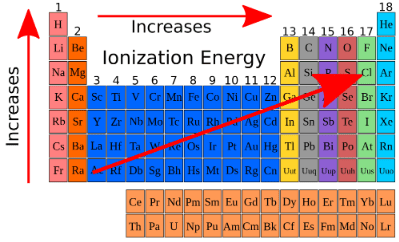Factors that influence Ionisation Energy

• Atomic size: the larger the size of the atom, the smaller the I.E. i.e., I.E. µ
• Effective nuclear charge: The greater the effective charge on the nucleus of an atom, the more difficult it would be to remove an electron from the atom because electrostatic force of attraction between the nucleus and the outermost electron increases. So greater energy will be required to remove the electron.
• Penetration effect of orbitals: The order of energy required to remove electron from s,p,d-and¦-orbitals of a shell is s>p>d>¦.
• Shielding or screening effect: Screening effect results in decrease of force of attraction between the nucleus and the outermost electron and lesser energy is required to separate the electron. Thus the value of I.P. decreases.
• Stability of half-filled and fully-filled orbitals: According to Hund's rule the stability of half filled or completely filled degenerate orbitals is comparatively high. So comparatively more energy is required to separate the electron from such atoms.

Successive Ionisation Energies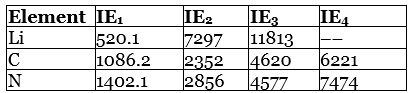3. Electron Affinity

Electron affinity increases along the period while decreases down the group.
Factors affecting the magnitude of electron affinity

• Atomic size – In general electron affinity value decreases with the increasing atomic radius because electrostatic force of attraction decreases between the electron being added and the atomic nucleus due to increase of distance between them.
• Effective nuclear charge – Electron affinity value of the element increase as the effective nuclear charge on the atomic nucleus increases because electrostatic force of attraction between the electron being added and the nucleus increases. As the electrostatic force of attraction increases, amount of energy released is more.
• Screening or Shielding effect – Electron affinity value of the elements decreases  with the increasing shielding or screening effect. The shielding effect between the outer electrons and the nucleus increases as the number of electrons increases in the inner shells.
• Stability of half filled and completely filled orbitals – The stability of half filled and completely filled degenerate orbitals of a sub shell is comparatively more, so it is difficult to add electron in such orbitals and lesser energy is released on addition of electron hence the electron affinity value will decrease.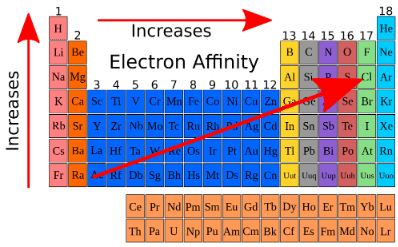Electron Affinities (kJ mol–1), M(g) + e– → M(g) + energy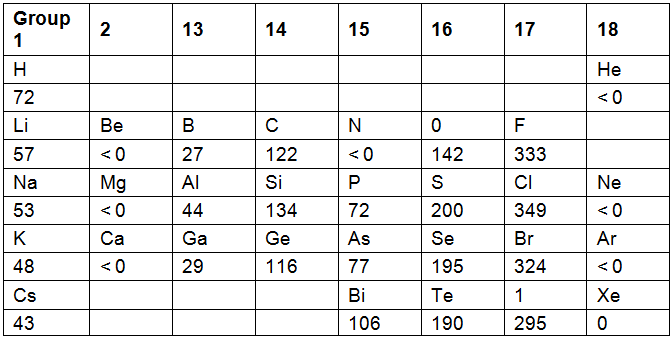4. Electronegativity

Electronegativity of elements increases along the period while decreases down the group.

Electronegativity scales

Some arbitrary scales for the quantitative measurement of electronegativities are as under

• Pauling's scale –If xA and xB are the electronegativities of atoms A and B respectivey then
0.208 √ΔAB= xA – xB if xA > xB
or ΔA= 23.06 (xA – xB)2
ΔAB = EA-B(experimental) – EA-B(theoretical)
where EA-B is the energy of A-B bond.
In a purely covalent molecule, AB, the experimental and theoretical values of bond energy A-B are equal.
So ΔAB = 0
or 0=23.06 (xA – xB)2
or x= xB
In an ionic molecule AB, EA-B(experimental) is more than EA-B(Theoretical).
Pauling assumed the electronegativity value of fluorine to be 4 and calculated the electronegativity values of other elements from this value.
• Mulliken's electronegativity: Electronegativitiy  = (Electron Afffinity - Ionization Potential)/2
when both are expressed in electron volt
• Alfred Rochow’s electronegativity:
If the distance between the circumference of outermost shell and the nucleus is r and the effective nuclear charge Zeff then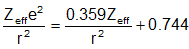Zeff = Z - σ
Z = The actual charge present on the nucleus i.e number of protons,
σ = Shielding constant

Factors affecting the magnitude of electronegativity

• Atomic radius: As the atomic radius of the element increases the electronegativity value decreases.
• Effective nuclear charge: The electronegativity value increases as the effective nuclear charge on the atomic nucleus increases.
• Oxidation state of the atom: The electronegativity value increases as the oxidation state (i.e. the number of positive charge) of the atom increases.
• Hybridisation state of an atom in a molecule: If  the s- character in the hybridisation state of the atom increases, electronegativity also increases.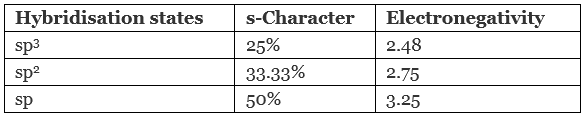5. Valency

It is the number of univalent atoms which can combine with an atom of the given element.

• Valency is given by the number of electrons in outermost shell.
• If the number of valence electrons ≤4: valency = number of valence electrons
• If the number of valence electrons >4: valency = (8 - number of valence electrons)
• Many elements exhibit variable valence (particularly transition elements and actinoids).
• Variation in a period − Increases from 1 to 4 and then decreases from 4 to zero on moving from left to right.
• Variation in a group − No change in the valency of elements on moving down a group. All elements belonging to a particular group exhibit same valency.?

6. Metallic Character of an Element

• Non-metallic elements have strong tendency to gain electrons.
• Non-metallic character is directly related to electronegativity and metallic character is inversely related to electronegativity.
• Across a period, electronegativity increases. Hence, non-metallic character increases (and metallic character decreases).
• Down a group, electronegativity decreases. Hence, non-metallic character decreases (and metallic character increases).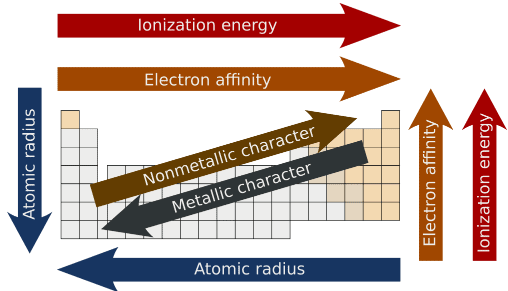The document JEE Notes | EduRev is a part of the JEE Course Chemistry for JEE.
All you need of JEE at this link: JEEUse Code STAYHOME200 and get INR 200 additional OFF Use Coupon Code
All Tests, Videos & Notes of JEE: JEE

### Top Courses for JEE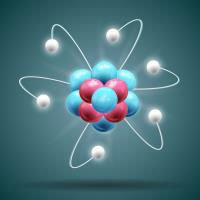## Chemistry for JEE

196 videos|450 docs|369 tests

### Top Courses for JEETrack your progress, build streaks, highlight & save important lessons and more!

,

,

,

,

,

,

,

,

,

,

,

,

,

,

,

,

,

,

,

,

,

;